2016-07-04 10:38:28 qq_26499769 阅读数 22242
• ###### MATLAB图像处理

全面系统的学习MATLAB在图像处理中的应用

20342 人正在学习 去看看 魏伟

2.3.3 赋范空间2.3.6 希尔伯特空间6.1.2   数字图像的傅立叶变换6.1.3  快速傅立叶变换的算法

FFT并不是一种新的变换，它只是傅立叶变换算法实现过程的一种改进。FFT中比较常用的是蝶形算法。蝶形算法主要是利用傅立叶变换的可分性、对称性和周期性来简化DFT的运算量。下面就来介绍一下蝶形算法的基本思想。6.4.3 主成分变换的实现6.4.4 基于K-L变换的图像压缩6.4.2 主成分变换的推导1.1.3 函数的极限1.3.2 内积与外积1.4.5   卷积定理及其证明1.1.2 级数的敛散1.1 极限及其应用

1.1.1 数列的极限1.3.7 曲面积分2.3.7 索伯列夫空间2.3.5 内积空间

前面我们已经讨论过关于内积的话题，此处以公理化的形式给出内积的定义。2.3.2 距离空间
尽管在线性空间上我们已经可以完成简单的线性运算，但这仍然不能满足我们的需求。为了保证数学刻画的精确性，还必须引入距离的概念。本文最初是从极限开始讲起的，它是因此微积分的必备要素之一，而极限的概念显然也是基于距离上无限接近这样一种角度来描述的。由此，在距离空间中，可以引入“任意逼近”的概念，即极限概念。一般来说，一个集合如果能够在其中确切地引入任意逼近的概念，就称之为“拓扑空间”。而距离空间是一种最常用的拓扑空间。

### 2.3  泛函与抽象空间

#### 2.3.1  线性空间2018-04-27 14:41:53 jayandchuxu 阅读数 350
• ###### MATLAB图像处理

全面系统的学习MATLAB在图像处理中的应用

20342 人正在学习 去看看 魏伟

# 矩阵的特征值、特征向量的概念

## 定义

Ax=λx(1)

|λEA|=0(2)$|\lambda E-A|=0 \tag{2}$
(λEA)x=0(3)$(\lambda E-A)x=0 \tag{3}$

|λEA|=λn(a11+a22++ann)λn1++(1)n|A|

λn(a11+a22++ann)λn1++(1)n|A|=0(4)

1. n$n$阶矩阵在复数范围内，一定有n$n$个特征值（重特征值按重数计算个数）。
2. n$n$阶矩阵在实数范围内有多少个特征值是不一定的
3. n$n$阶实对称矩阵可以看成是一个特例，因为它一定有n$n$个实特征值（重特征值按重数计算个数）。如果其中一个特征值λ=0$\lambda=0$，矩阵的秩r(A)=k$r(A)=k$，（0<k<n$0k$k$是正整数），则λ=0$\lambda=0$恰为A$A$nk$n-k$重特征值。
4. 如果 n$n$阶矩阵A$A$不是对称矩阵，那么，λ=0$\lambda=0$至少为A$A$nk$n-k$重特征值。

## 作用

A$A$是一个n×n$n\times n$方阵时，只涉及到旋转，拉伸。如果矩阵在实数域内可以得到n$n$个特征值（重特征值按重数计算个数），那么利用其对应的特征向量（单位化后）组成矩阵正交Q$Q$可以使得：

A=QΛQ1

# 正交矩阵

## 正交矩阵的性质

1. 正交矩阵的转置伴随矩阵、之间的积矩阵都是正交矩阵；
2. .每一行（列）都是单位向量
3. 任意两行或两列相互垂直
4. 其行列式等于±1

# SVD分解

## 根据奇异值分解

n×n$n\times n$阶矩阵按特征值分解相似，任一m×n$m\times n$阶矩阵A$A$也可以写成类似的形式：

A=UΣVT(5)

(ATA)vi=λivi(6)

δi=λi(7)

ui=1δiAvi(8)

# 用奇异值分解图像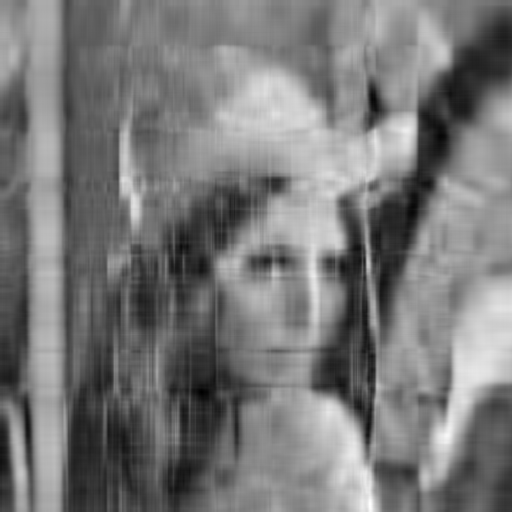## 代码

import cv2
import numpy as np
from numpy import linalg as la  # 用到别名
from scipy.misc import imsave

import scipy
print(im.shape)
gray = cv2.cvtColor(im, cv2.COLOR_BGR2GRAY)
U, Sigma, VT = la.svd(gray)
print('矩阵U的形状：', U.shape, '    矩阵Sigma的形状：',
Sigma.shape, '    矩阵VT的形状：', VT.shape)
se = np.eye(512, dtype=np.float64)
n = 512
i = 0
k = 30  # 保留特征值数目
# 改变特征值
while i < n:
if i > k - 1:
Sigma[i] = 0
se[i, i] = Sigma[i]
i += 1

svt = np.dot(se, VT)
usvt = np.dot(U, svt)
imsave('USVT_Sigma=30m.bmp', usvt)

# 参考资料

1. 秦川, 李小飞. 方阵的秩与特征值的关系[J]. 课程教育研究:学法教法研究, 2015(27):120-120.
2. 如何通俗易懂地解释「协方差」与「相关系数」的概念？
3. 如何理解矩阵特征值？马同学的回答
4. 矩阵的特征值分解与奇异值分解的几何意义
5. 杜美华, 孙建英. 正交变换的几何意义及其应用[J]. 哈尔滨师范大学自然科学学报, 2014, 30(3):36-39.

2013-09-26 23:21:17 F_SUNNY 阅读数 2167
• ###### MATLAB图像处理

全面系统的学习MATLAB在图像处理中的应用

20342 人正在学习 去看看 魏伟

从事图像处理这个行当也已经有一段时间了，对于一个自动化毕业的本科生，确实是磕磕绊绊的走到现在了，前期的基础图像处理还基本上能够轻松上手，越到后面的机器学习算法，用到的数学知识也就越深了，所以越到后面会越困难的。现在回想下之前的图像处理基础算法，其实是可以归纳到数学各个领域的。下面简单介绍下一些基础图像处理算法，当然主要是讲自己对其的归类理解，有不对的之处，大伙一起探讨。如果想要这些算法的具体实现，给推荐几个博客(百度的)，我也是从这些类似的博客起步的：

http://blog.csdn.net/xizero00/article/details/6631209

主要的基础图像处理算法有：灰度化，二值化，投影法，差影法，图像采样，增强，分割，边缘提取，匹配等。

下面讲讲这些算法最基础实现时按照数学知识归类：

1. 初等代数类：

①.灰度化：一般灰度化是用加权平均的，这个在我们小学都可以解决了，f(i,j)=0.30R(i,j)+0.59G(i,j)+0.11B(i,j))；

②二值化：其实二值化就是一个分段的0-1函数；

③差影法：这类的帧差发，前景提取法，都是一个减法，当然如果有背景建模之类的，那就背景建模的算法就属于高等代数或者概率统计的知识了；

④图像锐化：一般的图像锐化算法也是相邻像素点之间的初等代数运算，运算如下：

r = r1 + 0.25 * |r1 - r2|
g = g1 + 0.25 * |g1 - g2|
b = b1 + 0.25 * |b1 - b2|

⑤图像的叠加，浮雕效果等也都是属于初等代数的；

2.概率统计类：

①.投影法：像直方图统计之类的，是属于统计类的；

②.匹配算法：当然匹配算法也可以用线性代数，高等代数做，但是像方差匹配之类是属于概统的；

③图像采样：一听采样就知道是概统的，其中有图像区域采样，随机采样，分布采样，上/下采样；但是一般的采样算法都要结合代数知识进行应用，比如对图像感兴趣区域进行提取，可能还要用到插值算法进行归一化之类的；

3.高等代数：

①边缘提取：这主要用到的是一阶二阶微分，卷积等知识，这些都是高等代数中的基础；

②图像滤波：卷积滤波，高通滤波；

4.几何学：

5. 线性代数：

①PCA降维：这个是基础的人脸识别算法，很好的引用了矩阵的特征值，特征向量等知识，是经典算法；

②匹配：结合几何等特征，可以对图像进行线性匹配；

③非线性的线性化，通过类hogh等变换，可以将高维非线性数据，线性化进行处理，这样可以提高效率，简化算法。

2018-11-20 21:50:32 StephenArk 阅读数 360
• ###### MATLAB图像处理

全面系统的学习MATLAB在图像处理中的应用

20342 人正在学习 去看看 魏伟

# 4.2 机器学习、人工智能、图像处理、图像识别导论

## 4.2.0 前导知识

• 微积分（高数）
• 线性代数
• 概率论与统计
• 离散数学（用的没有上面三门多）

## 4.2.1 机器学习## 4.2.2 人工智能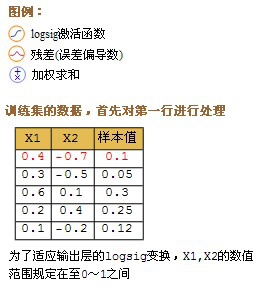$logsig(x) = \frac{1}{1+e^{-x}}$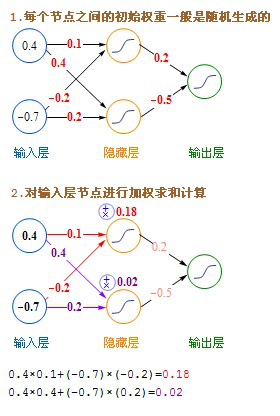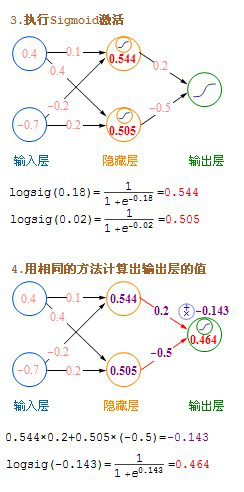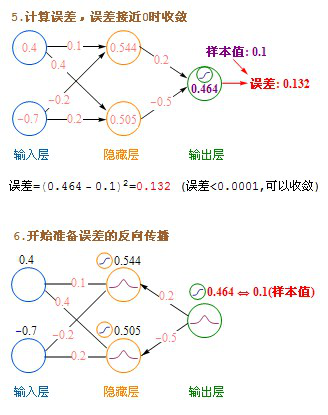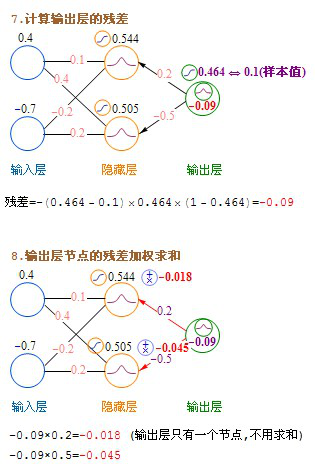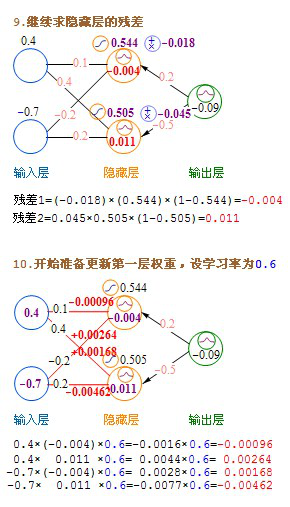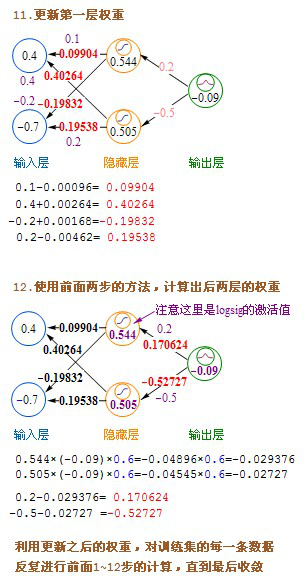## 4.2.4 图像识别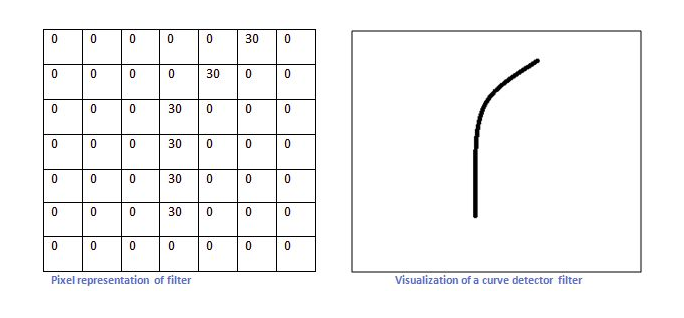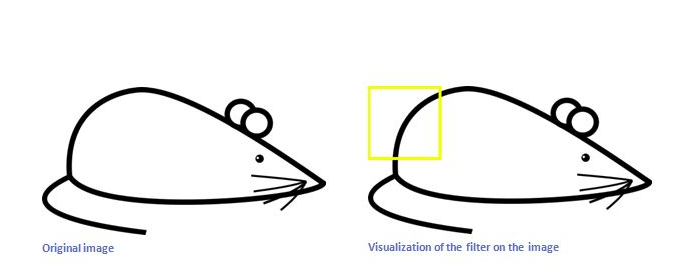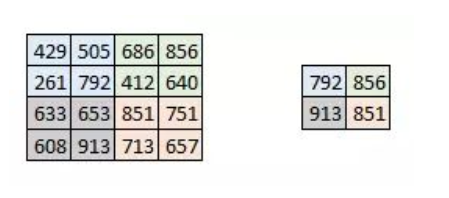2016-07-26 09:49:21 qq_19891827 阅读数 1227
• ###### MATLAB图像处理

全面系统的学习MATLAB在图像处理中的应用

20342 人正在学习 去看看 魏伟

（1）.HTML5 canvas getImageData() 方法;
getImageData() 方法返回一个 ImageData 对象，该方法下的data对象，以一维数组的方式拷贝了画布指定矩形的像素数据。

R - 红色 (0-255)
G - 绿色 (0-255)
B - 蓝色 (0-255)
A - alpha 通道 (0-255; 0 是透明的，255 是完全可见的)。参数 描述 x 开始复制的左上角位置的 x 坐标。 y 开始复制的左上角位置的 y 坐标。 width 将要复制的矩形区域的宽度。 height 将要复制的矩形区域的宽度

（2）.putImageData() 方法：

 参数 描述 imgData 规定要放回画布的 ImageData 对象。 x ImageData 对象左上角的 x 坐标。 y ImageData 对象左上角的 y 坐标。 dirtyX 水平值，在画布上放置图像的位置。 dirtyY 水平值（y）,在画布上放置图像的位置 dirtyWidth 在画布上绘制图像所使用的宽度 dirtyHeight 在画布上绘制图像所使用的高度

（1）黑白二值化：首先对图像进行黑白二值化处理，通过getImageData()方法获取画布中图像的所有像素的RGBA信息，以128为界，与data数组中每个RGBA值作对比，低于128则将其数值变为0，高于128将其数值变为255，经过此阶段处理后，图像中只保留黑、白两个颜色，图像的集合性质只与像素值为0或255的点的位置有关，不再涉及像素的多级值，使处理变得简单，而且数据的处理和压缩量小。下图（左）为原图，下图（右）为经黑白二值化处理后的图像。var cvs=document.getElementById('canvas');
var HEIGHT=cvs.height;
var WIDTH=cvs.width;
var ctx=cvs.getContext('2d');
var pixel = null;
var opixel=null;
var xpixel=null;
var edge=[];
var img=new Image();
img.src='http://ui.yidaochn.com/test/zz.jpg';
ctx.drawImage(img,0,0,WIDTH,HEIGHT);
pixel = (ctx.getImageData(0, 0, WIDTH, HEIGHT));
whiteBlack();
};

//黑白二值化
function whiteBlack(){
var red=0;
var green=0;
var blue=0;
var index=null;
var average=null;
for(var i=0;i<HEIGHT;i++) {
for (var j = 0; j < WIDTH; j++) {
index=(pixel.width*i+j)*4;
red=index;
green=index+1;
blue=index+2;
average=Math.round((pixel.data[red]+pixel.data[green]+pixel.data[blue])/3);
if(average>=128){
pixel.data[red]=255;
pixel.data[green]=255;
pixel.data[blue]=255;
}else{
pixel.data[red]=0;
pixel.data[green]=0;
pixel.data[blue]=0;
}
}
}
ctx.putImageData(pixel,0,0);
}

（2）边缘查找：经过黑白二值化处理后的图像仅保留两种颜色（黑、白），对存有该图像RGBA值信息的data数组进行遍历，每个像素点上的RGB值一定为黑色（0,0,0）或白色（255,255,255）。所以这里我们仅仅比较每一像素点上R值（红色值）与其上、下、左、右相邻像素点的R值是否相等即可，如改点与上下左右4个点的R值相同说明该点不是图像图形的边缘，如该点与至少上下左右4个点其中一个点R值不同，则说明该点为图形边缘，以此类推遍历所有颜色值数据即可得到图像边缘。

function imageEdge(){
opixel=ctx.getImageData(0,0,WIDTH,HEIGHT);
var red=0;
var index=null;
var redVal=null;
var prevRedVal=null;
var nextRedVal=null;
var topRedVal=null;
var bottomRedVal=null;

for(var i=0;i<HEIGHT;i++){
for(var j=0;j<WIDTH;j++){
index=(pixel.width*i+j)*4;
red=index;
redVal=pixel.data[red];
prevRedVal=red-4>=0?pixel.data[red-4]:pixel.data[red];
nextRedVal=pixel.data[red+4];
topRedVal=(red-WIDTH*4)>=0?pixel.data[red-WIDTH*4]:pixel.data[red];
bottomRedVal=pixel.data[red+WIDTH*4];

if(redVal!=nextRedVal||redVal!=topRedVal||redVal!=prevRedVal||redVal!=bottomRedVal){
opixel.data[red]=255;
edge.push(red);
}
}
}
ctx.putImageData(opixel,0,0);
}